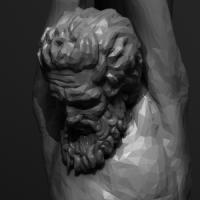1.## Librarian

Members

2

• ### Content count

1,378

2.## abvfx

Members

1

• ### Content count

601

3.## Noobini

Members

1

• ### Content count

2,032

4.Members

1

89

## Popular Content

Showing most liked content on 09/09/2021 in all areas

1. 2 points
newton's law and try to use this it works function float Kernel(vector r ; float h){ float q = length(r); q /= h; float factor = 1.0f / (PI * h * h * h ); if( q>0 && q<=1){ float right = 1.0f - 1.5f * (q * q) * (1.0f - q/ 2.0f) ; return factor * right; } if(q >1 && q <=2 ) { float left = factor / 4.0f; float right = pow(2.0f - q , 3); return left*right; } if(q>2) { return 0; } return 0; } function vector GradKernel(vector r; float h){ float q = length(r); q /= h; vector dir = normalize(r); float factor = 1.0f / (PI * h * h * h ); vector retgrad = set(0,0,0); if( q<=1){ retgrad = dir * (factor / h) * (-3.0 * q + 2.25f * q*q); } if( q<2){ retgrad = dir * (-0.75f * (factor / h) * pow((2.0f - q),2) ); } return retgrad; } float h = chf("h"); @P.y = Kernel(@P, h); @N = GradKernel(@P, h); NewtonsLaw.hiplc
2. 1 point
my H19 wish list. I just wish for it to be released
3. 1 point